Get instant live expert help with Excel or Google Sheets“My Excelchat expert helped me in less than 20 minutes, saving me what would have been 5 hours of work!”

#### Post your problem and you’ll get Expert help in seconds.

Your message must be at least 40 characters
Our professional Expert are available now. Your privacy is guaranteed.

# VLOOKUP from Another Sheet

In Excel, we usually have to work with more than one worksheet. The VLOOKUP function is able to be used on another sheet which allows the ability to better organize our data and perform calculations on it. This tutorial will assist all levels of Excel users in performing a VLOOKUP from another sheet.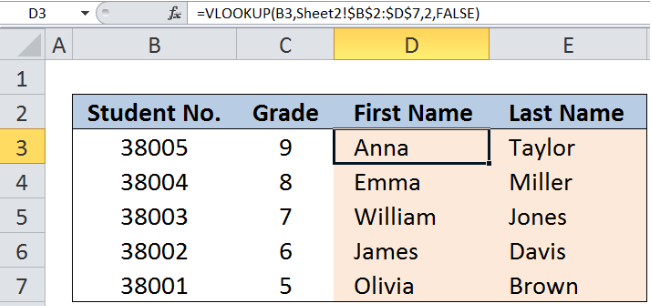Figure 1.  Final result: VLOOKUP from another sheet

Working formula: `=VLOOKUP(B3,Sheet2!\$B\$2:\$D\$7,2,FALSE)`

## VLOOKUP in Excel

VLOOKUP is used when we want to look up and retrieve data from a given data set.  To look up from another sheet, the syntax is:

### Syntax

`=VLOOKUP(lookup_value, sheet_range, col_index_num, [range_lookup])`

Where

• Lookup_value: the value we want to search and find in another worksheet
• Sheet_range:    the range of cells in another worksheet containing the data we want to retrieve
• Col_index_num: the column number in the sheet_range corresponding to the information we want to retrieve, relative to the first column
• [range_lookup]: optional; value is either TRUE or FALSE;
• if TRUE or omitted, VLOOKUP returns either an exact or approximate match; it is important to sort the first column of the table_array in ascending order to ensure that VLOOKUP returns the correct value
• if FALSE, VLOOKUP will only find an exact match.

## Setting up the Data

Here we have an Excel file with 2 worksheets.

Sheet1 has a list of student numbers and corresponding grade levels, but with missing first and last names.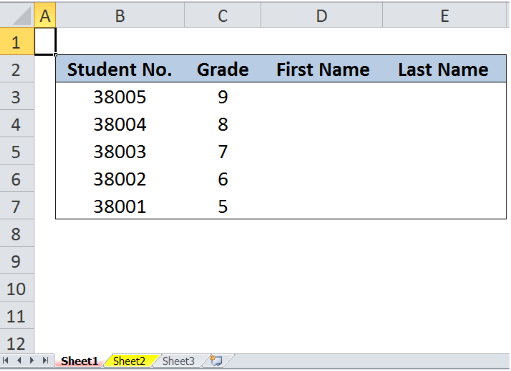Figure 2. Sample data in Sheet1 with missing first and last names

In Sheet2, we have a list of student numbers and corresponding first and last names.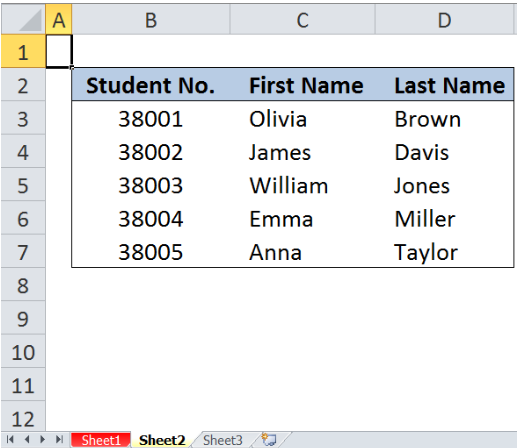Figure 3. Sample data in Sheet2 with complete names per student number

## VLOOKUP from Sheet2

We want to populate columns D (First Name) and E (Last Name) in Sheet1 with the data in columns C (First Name) and D (Last Name) from Sheet2.

### Retrieving the First Name

In cell D3, enter the formula:

`=VLOOKUP(B3,Sheet2!\$B\$2:\$D\$7,2,FALSE)`

Where:

• B3 is the lookup value, which is the student number; it is the common value between the two sheets that we will use to retrieve the information from Sheet2 to Sheet1
• Sheet2!B2:D7 is the range of data in Sheet2 where we want to search for the student number, in order to retrieve the first names and last names
• The dollar symbols “\$” in \$B\$2:\$D\$7 fix the data range to make it easier to copy and paste the formula to other cells later
• 2 as the col_num_index means that we want to retrieve the value in the second column of the data range B2:D7, which is the First Name (under column C)

The formula returns the first name Anna for student number 38005.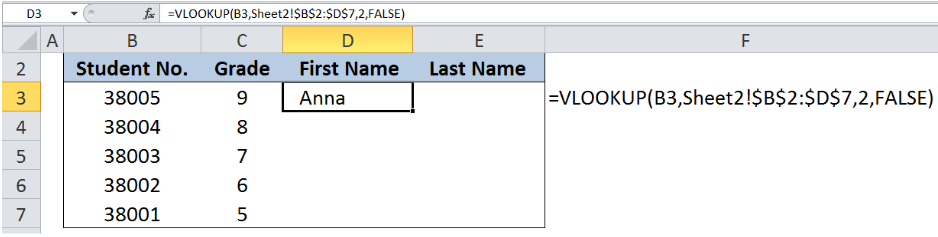Figure 4.  Entering the VLOOKUP formula for first name from another sheet

### Retrieving the Last Name

In cell E3, enter the formula:

`=VLOOKUP(B3,Sheet2!\$B\$2:\$D\$7,3,FALSE)`

This is a similar formula, only this time, the col_num_index used is 3, which means that we want to retrieve the data in the third column of the data range B2:D7 in Sheet2, which is column D, containing the Last Name.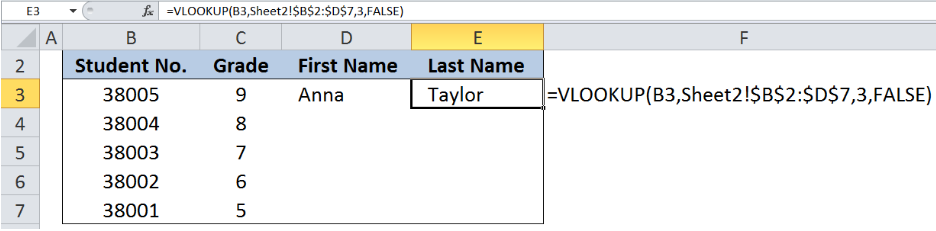Figure 5. Entering the VLOOKUP formula for last name from another sheet

To complete the first names and last names, enter the formula as shown in the tables below: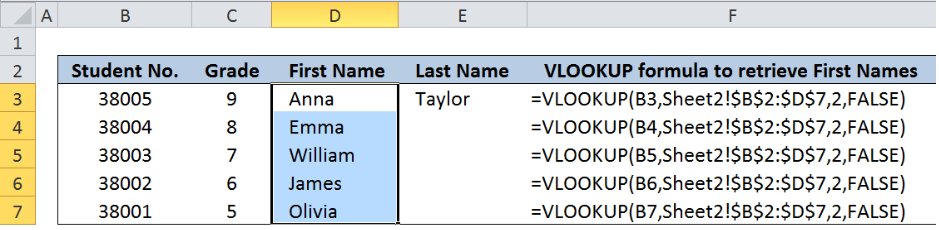Figure 6. VLOOKUP from another sheet for first names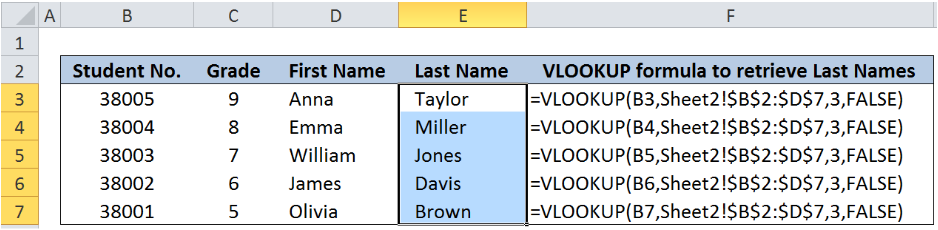Figure 7. VLOOKUP from another sheet for last names

Now we have successfully populated columns D and E in Sheet1, by using VLOOKUP to retrieve data from Sheet2.

Most of the time, the problem you will need to solve will be more complex than a simple application of a formula or function. If you want to save hours of research and frustration, try our live Excelchat service! Our Excel Experts are available 24/7 to answer any Excel question you may have. We guarantee a connection within 30 seconds and a customized solution within 20 minutes.

Are you still looking for help with the VLOOKUP function? View our comprehensive round-up of VLOOKUP function tutorials here.

Solution examplesI filtered data in column A (it is labeled ID #) of a data set. On my next worksheet, column A is also the ID #, but it is a different data set. I want to filter the ID #'s the same for the two sheets, but how do I do that being that I am working with two different sets of data?
Solved by V. H. in 22 minsI'm looking for a formula or format for if there is data in c4 on a sheet... It adds extra information underneath
Solved by X. E. in 20 minshi, my vlookup wont work in some rows but does in others. its temperemental. e.g it will work on rows 10 to 15, then not row 16 and 17, but the will for the rest.
Solved by K. Q. in 21 minsUse the Vlookup Function to complete the "employee" column of table 2. Use "job Id" from table 2 as your lookup_value(s) and table 1 as your reference.
Solved by C. H. in 16 minsIf a cell in another sheet is populated I need a vlookup done. If the cell is not populated I need the cell to return blank.
Solved by T. D. in 60 mins